# Math Practice Topic: Trapezoids

 Description: Perimeter and area problems with trapezoids. Adaptive Learning Progression: The way in which you must analyze the trapezoid becomes more advanced. Start using MathScore for free

## Sample Levels (out of 5)

Find the perimeter and area.

 1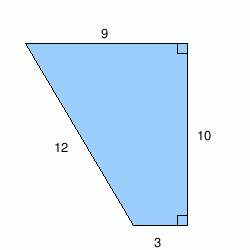perimeter = units area = units2

Find the perimeter and area.

 1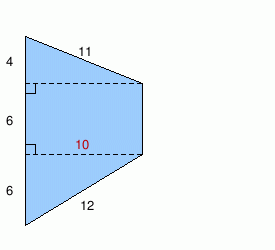perimeter = units area = units2 2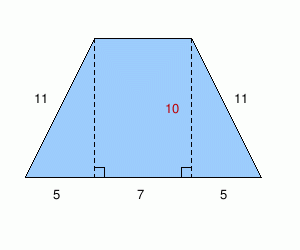perimeter = units area = units2

Find x.

 1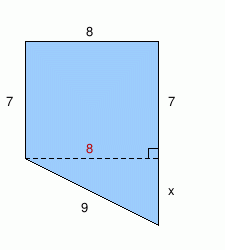If the area is 72 units2, then what is x? x = units 2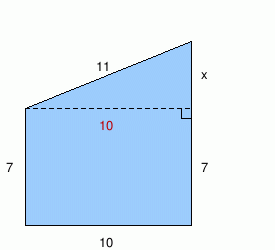If the area is 90 units2, then what is x? x = units